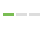# 【leetcode】5. Longest Palindromic Substring最长回文子串## 解法1：中心拓展算法

### 实现代码

``````class Solution:
def longestPalindrome(self, s: str) -> str:
n = len(s)
Max,sub = 0,s[0:1]
for i in range(n):
tmp = self.searchPalindrome(i-1,i+1,s)
if len(tmp) > Max:
Max = len(tmp)
sub = tmp
tmp = self.searchPalindrome(i-1,i,s)
if len(tmp) > Max:
Max = len(tmp)
sub = tmp
return sub

def searchPalindrome(self, left: int, right: int, s: str) -> int:
sub = ""
while left != -1 and right != len(s):
if s[left] == s[right]:
sub = s[left:right+1]
left-=1
right+=1
else : break
return sub``````

### 马拉车算法

``````class Solution:
# Manacher 算法
def longestPalindrome(self, s: str) -> str:
# 特判
if len(s) < 2 or s == s[::-1]:
return s

# 得到预处理字符串
t = "#" + "#".join(s) + "#"

# 新字符串的长度
t_len = len(t)

# 数组 p 记录了扫描过的回文子串的信息
p = *t_len

# 双指针，它们是一一对应的，须同时更新
max_right = 0
center = 0

# 当前遍历的中心最大扩散步数，其值等于原始字符串的最长回文子串的长度
max_len = 1
# 原始字符串的最长回文子串的起始位置，与 max_len 必须同时更新
start = 1

for i in range(t_len):
if i < max_right:
mirror = 2 * center - i
# 这一行代码是 Manacher 算法的关键所在，要结合图形来理解
p[i] = min(max_right - i, p[mirror])

# 下一次尝试扩散的左右起点，能扩散的步数直接加到 p[i] 中
left = i - (1 + p[i])
right = i + (1 + p[i])

# left >= 0 and right < t_len 保证不越界
# t[left] == t[right] 表示可以扩散 1 次
while left >= 0 and right < t_len and t[left] == t[right]:
p[i] += 1
left -= 1
right += 1

# 根据 max_right 的定义，它是遍历过的 i 的 i + p[i] 的最大者
# 如果 max_right 的值越大，进入上面 i < max_right 的判断的可能性就越大，这样就可以重复利用之前判断过的回文信息了
if i + p[i] > max_right:
# max_right 和 center 需要同时更新
max_right = i + p[i]
center = i

if p[i] > max_len:
# 记录最长回文子串的长度和相应它在原始字符串中的起点
max_len = p[i]
start = (i - max_len) // 2
return s[start: start + max_len]``````

### 成果继续浏览关于 的文章

本文最后更新于：2020/02/06 14:43:58，可能因经年累月而与现状有所差异

• 浏览总量：64554 次
• 网站运行：1325 天
• 上次在线：7小时前
• 最后更新：7小时前
• 加载耗时：0.04 s
• 使用内存：3.72 MB
• 当前在线：5 人